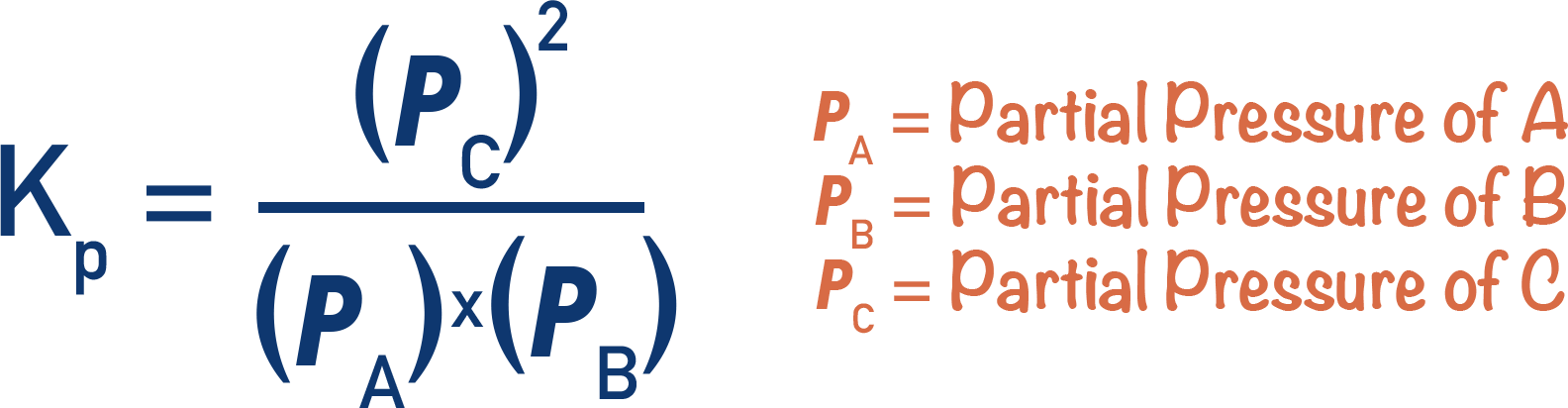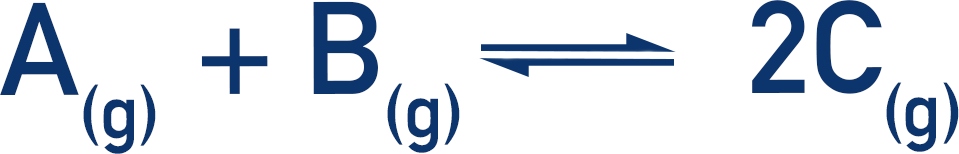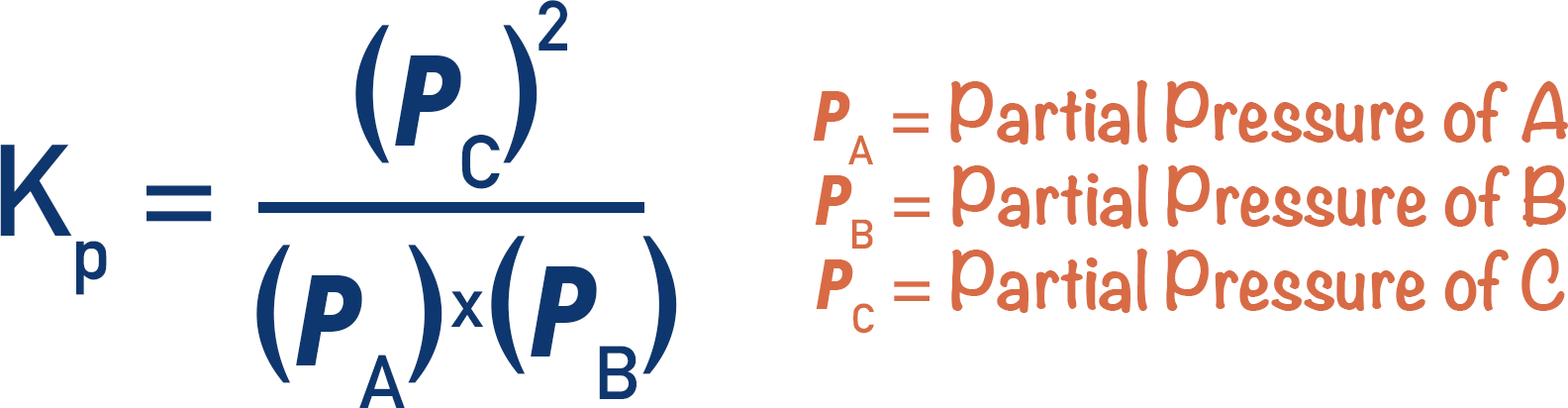Quick Notes Equilibrium Constant, Kp

• The equilibrium constant, Kp, describes the ratio of reactants compared to products at equilibrium for a gaseous equilibrium system.
• Kp is found by dividing the partial pressures of all products by the partial pressures of all reactants (raised to the power of their molar ratios).• The partial pressures of a gas in a closed system are directly linked to the moles of that gas.
• Partial pressures of reactants and products can be used to determine how much the forward or reverse reaction is favoured.

Full Notes Equilibrium Constant, Kp

The equilibrium constant, Kc, is a constant used to describe how much the forward or backward reaction is favoured in an equilibrium system. .

The equilibrium constant, Kp, works in the same way as Kc. The key difference is that Kc uses molar concentrations (mol dm-3), whereas Kp uses partial pressures.

For the following reaction:By taking known moles of gases and the total pressure of a system, Kp can be found for a particular reversible reaction. For how to find mole fractions and partial pressures, see Mole Fractions and Partial Pressures.Be aware when using Kp expressions to check units! Kp can have different units for different systems, also make sure to check that units used for pressure are constant (Pa and kPa).